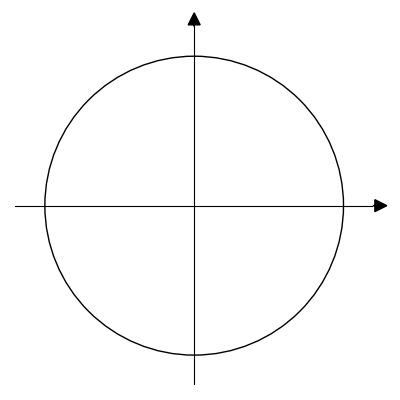# Drawing Trigonometric Axes in Python

Again one of these posts where I wonder how many people really need do to what I am about to explain, but who knows?

#### Let’s say you want to draw just the trigonometric circle and axes

1. Copy the TrigAxes() class from my GitHub notebook
```# Create the trigonometric axis, you can change the figuresize
axes = TrigAxes(figsize=(4,4))

# Just to plot the trigonometric axes
axes.draw()

# You can also save them as an image
axes.draw(imgpath="trig_circle.png")```#### Now let’s say you also want to add angles

1. Copy the TrigAxes() and the Angle() class from my GitHub notebook
```# Create the trigonometric axis
axes = TrigAxes(figsize=(5,4))

# Add as many different angles as you like to the axes
axes.addAngle(Angle(30, label='theta1 = 30', color='blue', linewidth=0.02))
axes.addAngle(Angle(-30, label='theta2 = -30', color='red', linewidth=0.02))

axes.draw(imgpath="circle_angles.png")
```